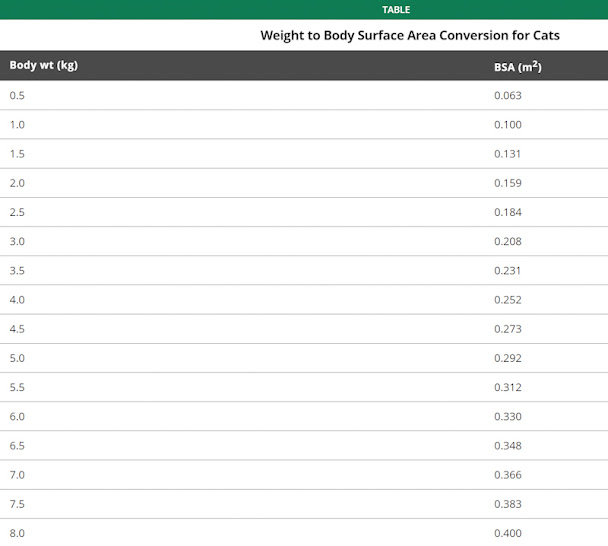### How many hairs on a domestic cat?

CONCLUSION: 68,250,000 hairs on a standard-sized cat weighing 10 pounds.

I have tried to work this out before. Perhaps it would be easier to simply read what somebody else has calculated. It is very hard to be accurate because the geometry of the domestic cat means that it is very difficult to apply some basic formulas to work out the surface area. My research indicates that there are about 60,000 pairs per square inch or 25,000 hairs per square centimetre on a normal cat.I decided to work out the number of hairs on the torso of a cat which is somewhat cylindrical and then add in the legs and head and tail. Fortunately, there is an online calculator to work out the surface area of a cylinder.

### Smaller or standard domestic cat

I have found a veterinary chart on the MSD Veterinary Manual website which converts cat weight to surface area. A 10 pounds cat weighs 4.54 kilograms with a surface area of 0.273 square meters or 2730 square centimeters. Multiplying 2730 x 25,000 makes 68,250,000 hairs on a 10 pound cat.Chart showing the surface area of cats of different body weights. Source: see above.

### Large domestic cat!

I worked this out from scratch because I had not found the above chart at the time.

If we say that the length of the torso of a cat is 45 cm and the radius of this imaginary cylinder is 12.7 cm, the total surface area works out at 4662 cm². If we multiply that number by 25,000 (the number of hairs per square centimetre on a normal) we come to the grand total of 116,550,000 hairs on a standard domestic cat excluding the head, legs and tail.

I'm going to estimate that the area of all four legs is about 250 cm². The head has a radius of about 5 cm and if we take the head to be a sphere, which is not but it is near as I can get when working out the surface area, my online calculator makes that 314 cm². If we add that to the legs, we get 564 cm² which we then multiply by 25,000 to make 14,100,000. We then add that to the number of hairs on the torso to make 130,650,000 hairs on a normal cat's body.

I am now going to try and measure my cat to make sure that the length of his torso is as I have stated! Result: my cat is about 35 cms long on the torso. Can't measure the radius! Surprised?